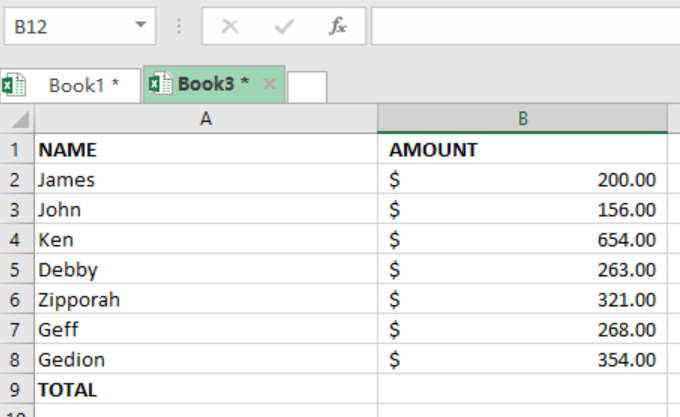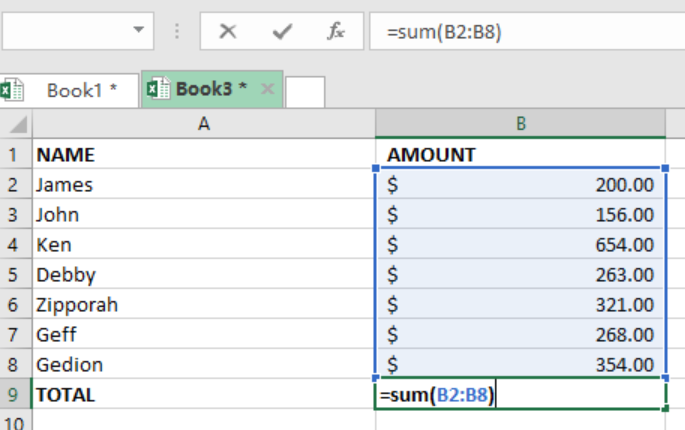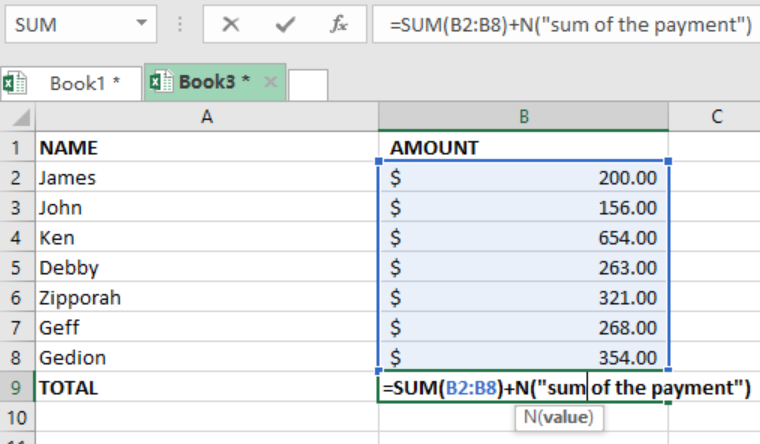Get instant live expert help with Excel or Google Sheets“My Excelchat expert helped me in less than 20 minutes, saving me what would have been 5 hours of work!”

#### Post your problem and you’ll get Expert help in seconds.

Your message must be at least 40 characters
Our professional Expert are available now. Your privacy is guaranteed.

Many at times we may need to leave a comment in a formula without disrupting or affecting the operation of the formula. While this can be hard for most people, here is a step by step guide on how to do so.

Note that for documentation purposes, you will need to leave comments in formula while working with excel spreadsheets. This is important as the comments will help you know what the functions are for, or specific instructions regarding the formula. Below is how this is done:

## Formula

`=Formula+ N(“comment”)`

The N function can be used to leave a comment in a formula which returns a numeric result. In our formula above, the N() allows us to leave an in-cell comment.

It is important to remember that the N function will only work if you use a numeric value, as it will return a number. But if you use a text value, then the function will return a zero as the result.

When the cell in which the formula has been applied is selected, the comment shall be seen in the formula bar.

## Example 1Figure 1. Setting up dataFigure 2. Input formula

Step 3: Add the N() FunctionFigure 3. Add the N() Function

## Note:

You must remember to use the quotation marks inside the N() function for you to get the correct result.

Most of the time, the problem you will need to solve will be more complex than a simple application of a formula or function. If you want to save hours of research and frustration, try our live Excelchatservice! Our Excel Experts are available 24/7 to answer any Excel question you may have. We guarantee a connection within 30 seconds and a customized solution within 20 minutes.

### Did this post not answer your question? Get a solution from connecting with the expert.Another blog reader asked this question today on Excelchat:
Solution examplesi want to hide a column but still use it in formula. is this even possible?
Solved by X. E. in 11 minsC5 contains 1625MPGW, F5 contains quantity. Need to search for MPGW and have quantity insert into column H5.
Solved by E. C. in 33 minsNeed help with lookup when you have multiple lookup values
Solved by V. J. in 20 minsI have a spread sheet with Items and sub Items. I would like to know if I can place a letter in another column it can get it to list the sub item number on another page in order
Solved by S. H. in 60 minsNeed to seperate text thats in a cell so i can sum them
Solved by G. C. in 10 mins## Subscribe to Excelchat.coAnother blog reader asked this question today on Excelchat: